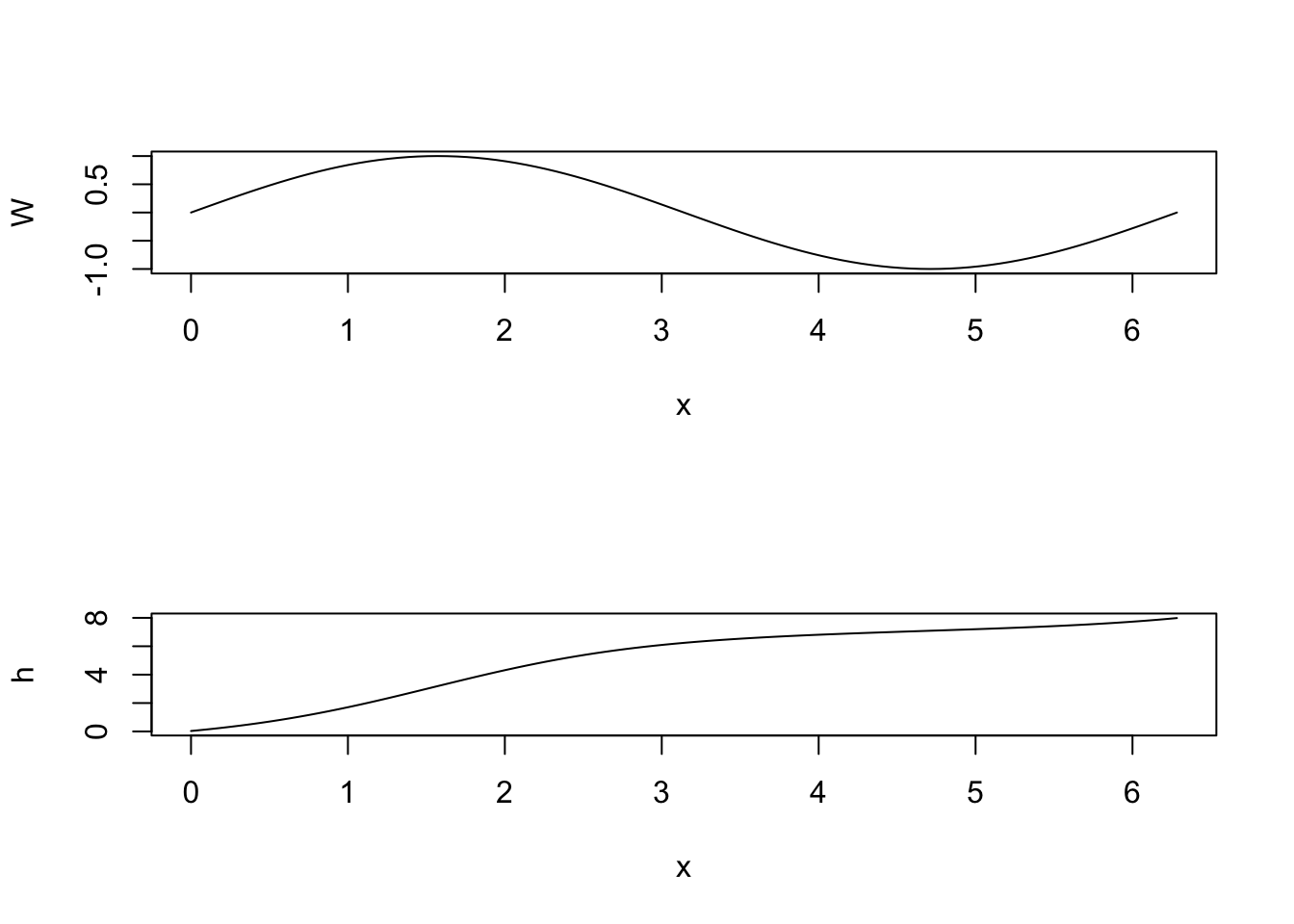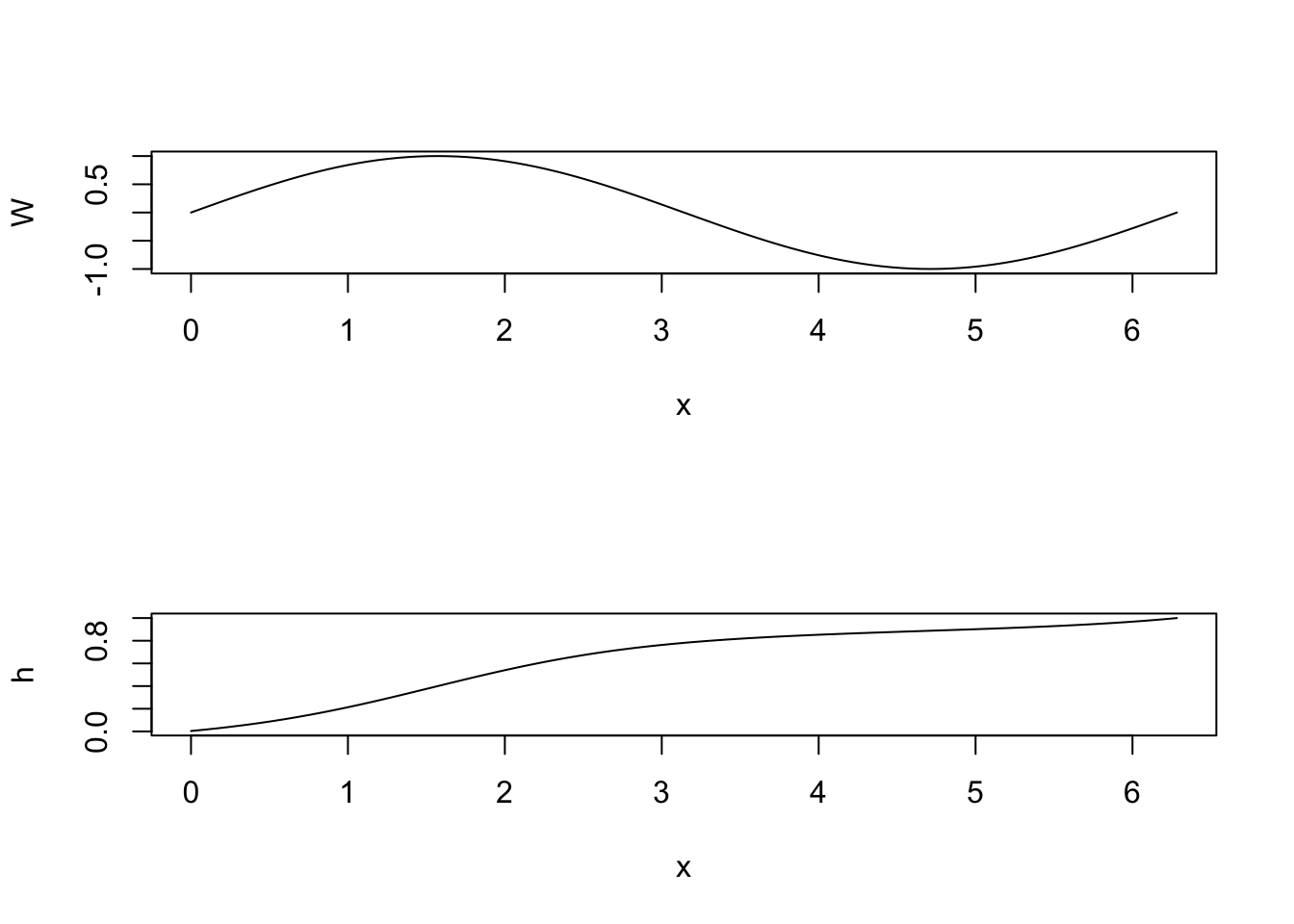library(fda)

## R Markdown

Let $$x$$ be a value of a strictly monotone function $$h(x)$$. Let $$W(x)$$ be an arbitrary unconstrained function. We can turn this function into a strictly monotone function by first exponentiating it to make a positive version, and then integrating the positive function from an origin value to the desired value $$x$$:

$h(x) = \int_0^x \exp[W(u)] du$

For example, let’s make $$W(x) = \sin(x)$$ and do the integration from 0 to $$2 \pi$$.

# create a fine mesh of x-values over [0,2*pi]
x <- seq(0,2*pi,len=101)
# step size
delta <- 2*pi/100
# sin(x)
W <- sin(x)
# exponentiate the result
EW <- exp(W)
# compute the integral from 0 to 2*pi using the trapezoidal rule
hof2pi <- delta*(sum(EW) - EW/2)
print(paste("h(2*pi) =",round(hof2pi,2)))
#>  "h(2*pi) = 7.99"

Now display the complete function $$h(x)$$ from 0 to $$2 \pi$$ along with $$W(x)$$.

h <- delta*(cumsum(EW) - EW/2)
par(mfcol=c(2,1))
plot(x, W, type="l")
plot(x, h, type="l")Notice that, near $$W(\pi/2) = 1$$, $$h$$ is increasing the most rapidly, and that where $$W$$ is negative the slope of $$h$$ is much closer to zero.

Often we want $$h(x)$$ to end up at a fixed point. Let’s use 1, for example. Then we simply divide $$h(x)$$ by $$h(2 \pi)$$.

h <- delta*(cumsum(EW) - EW/2)/hof2pi
par(mfcol=c(2,1))
plot(x, W, type="l")
plot(x, h, type="l")In applications, we often need to define a general function $$W$$ that can take any shape. Our goal may be, for example, to pass a smoothly increasing curve through a set of points with an increasing trend, but not necessary increasing from one value to the next.

We can do this by defining a B-spline basis with enough basis functions to permit any shape that we might need. The function $$W(x|c_1,\ldots,c_K) = \sum_{k=1}^K c_k \phi_k(x)$$ where $$\phi_k(x)$$ is a B-spline basis function. We can then optimize the fit to the data by minimizing sum of squared errors with respect to the $$K$$ coefficients $$c_k$$. Two functions in the fda package that do this are smooth.monotone() and smooth.morph().

The first of these has no restriction on the end point of the curve, and the second fits a curve that ends at a specified value. For example, we can use smooth.morph() to estimate a cumulative distribution function that begins at 0 on the left, ends at 1 on the right and is strictly monotonic and also smooth.